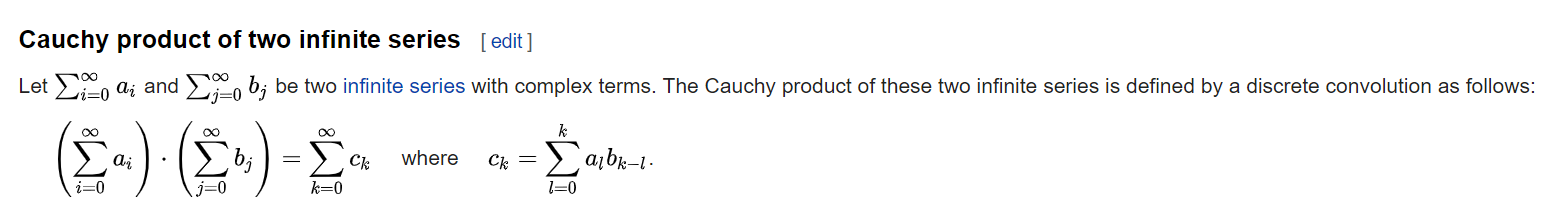Week 3 of the first phase of this coding period is coming to an end. The week started with a bad note, given that I had fallen sick due to travelling. But the work in Week 2, and the meeting with Sartaj on 11th of June helped me to progress smoothly. This week was spent mostly in completing the following deliverables.

• This week was spent mostly in deciding the ways to implement the various operations in `Formal Power Series`. I started with `convolution` since I felt that was the only operation which didn’t require a tough recursive implementation. `Discrete convolution`, or specifically `Cauchy Product` of two infinite series goes as following –Keeping this in mind, Sartaj and I decided to first make a boiler-plate function `convolve` which will take in two functions, `f(x)` and `g(x)` and an `order` term and will return the convolved formal power series, truncated upto the order term. I then came up with PR #17017, which does this following operations –

``````>>> f1, f2 = fps(sin(x)), fps(exp(x))
>>> f1.convolute(f2, x, order=6)
x + x**2 + x**3/3 - x**5/12 + O(x**6)
``````

Note that no `FormalPowerSeries` object is being returned as of now. We will deal with that in the upcoming PR.

• After pushing in the PR, Sartaj suggested to turn all the implementations in the `convolve` functions inot sequence-based implemetations, since `Sequences` in general should also cache the coefficients and speed for later calls as well. I used the `convolution` function in `sympy.discrete.convolutions` and have implemented `linear`, `cyclic`, `dyadic` and `subset` convolution as of now in `convolve` function of formal power series. Some examples are –
``````>>> from sympy import fps, sin, exp, convolution
>>> from sympy.abc import x
>>> f1 = fps(sin(x))
>>> f2 = fps(exp(x))

>>> f1.convolve(f2, x, 4)
x + x**2 + x**3/3 + O(x**4)

>>> f1.convolve(f2, x, 4, cycle=4)
11*x/12 + 35*x**2/36 + x**3/3 + O(x**4)

• In the meeting with Sartaj, we also discussed how we can decide upon the implementation of `composition` and `inversion` of formal power series. We decided that we should create a `FiniteFormalPowerSeries` class inherited from `FormalPowerSeries` and use that for implementing the various operations, so that common functions can be re-used. Soon, I will be implementing a `PR` for the above mentioned topics.